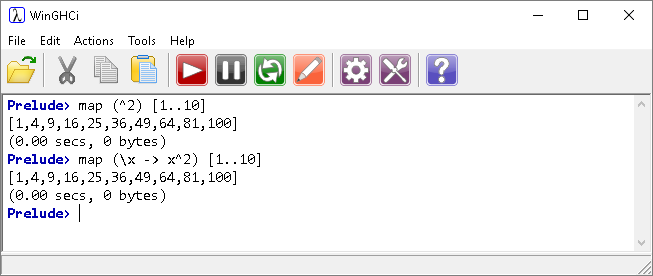# Introduction To Haskell Lambda Function

## Introduction

A lambda is an anonymous function made to be used only once in a program. Here are two ways of generating the same list,The second statement uses a lambda, \x. Lambda functions must start with a backslash. The lambda here represents the function that will be mapped to the list. The right-pointing 'arrow' defines that function. The x without a backslash represents the value taken from the list.

The lambda function in this case is completely unnecessary and does not make the code more readable to anyone who understands how the map function actually works.

There are times when using a lambda seems helpful. For example,In this case, the lambda has to evaluate to True or False.

Save and load a program consisting of this,

```z = filter (p) ["aa", "abc", "aaa", "abcd"]
where p x = length x > 2
```

Type z and press enter for the same result.

This could also be achieved by defining the p function separately. Using the where statement allows us to define an inline function. That funciton only has meaning in the definition of the z function.